#### Specialized algorithms

Now upper bounds are summarized for some narrower problems, which can be solved more efficiently than the general problem. All of the problems involve either two or three degrees of freedom. Therefore, we expect that the bounds are much lower than those for the general problem. In many cases, the Davenport-Schinzel sequences of Section 6.5.2 arise. Most of the bounds presented here are based on algorithms that are not practical to implement; they mainly serve to indicate the best asymptotic performance that can be obtained for a problem. Most of the bounds mentioned here are included in .

Consider the problem from Section 6.2, in which the robot translates in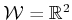and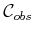is polygonal. Suppose that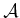is a convex polygon that hasedges and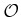is the union of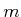disjoint, convex polygons with disjoint interiors, and their total number of edges is. In this case, the boundary of(computed by Minkowski difference; see Section 4.3.2) has at most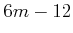nonreflex vertices (interior angles less than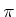) and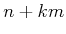reflex vertices (interior angles greater than). The free space,, can be decomposed and searched in time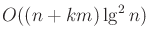[518,865]. Using randomized algorithms, the bound reduces to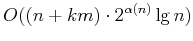randomized expected time. Now suppose thatis a single nonconvex polygonal region described byedges and thatis a similar polygonal region described byedges. The Minkowski difference could yield as many as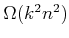edges for. This can be avoided if the search is performed within a single connected component of. Based on analysis that uses Davenport-Schinzel sequences, it can be shown that the worst connected component may have complexity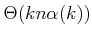, and the planning problem can be solved in time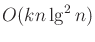deterministically or for a randomized algorithm,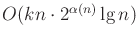randomized expected time is needed. More generally, ifconsists ofalgebraic curves in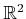, each with degree no more than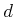, then the motion planning problem for translation only can be solved deterministically in time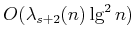, or with a randomized algorithm in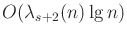randomized expected time. In these expressions,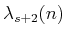is the bound (6.37) obtained from the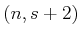Davenport-Schinzel sequence, and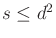.

For the case of the line-segment robot of Section 6.3.4 in an obstacle region described withedges, an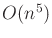-time algorithm was given. This is not the best possible running time for solving the line-segment problem, but the method is easier to understand than others that are more efficient. In , a roadmap algorithm based on retraction is given that solves the problem in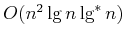time, in which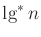is the number of times that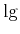has to be iterated onto yield a result less than or equal to(i.e., it is a very small, insignificant term; for practical purposes, you can imagine that the running time is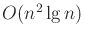). The tightest known upper bound is. It is established in  that there exist examples for which the solution path requires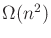length to encode. For the case of a line segment moving in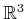among polyhedral obstacles with a total ofvertices, a complete algorithm that runs in time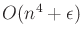for any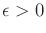was given in . In  it was established that solution paths of complexity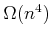exist.

Now consider the case for which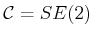,is a convex polygon withedges, andis a polygonal region described byedges. The boundary ofhas no more than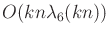edges and can be computed to solve the motion planning problem in time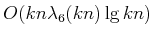[10,11]. An algorithm that runs in time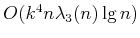and provides better clearance between the robot and obstacles is given in . In  (some details also appear in ), an algorithm is presented, and even implemented, that solves the more general case in whichis nonconvex in time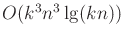. The number of faces ofcould be as high as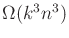for this problem. By explicitly representing and searching only one connected component, the best-known upper bound for the problem is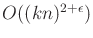, in whichmay be chosen arbitrarily small .

In the final case, suppose thattranslates in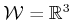to yield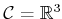. For a polyhedron or polyhedral region, let its complexity be the total number of faces, edges, and vertices. Ifis a polyhedron with complexity, andis a polyhedral region with complexity, then the boundary ofis a polyhedral surface of complexity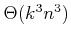. As for other problems, if the search is restricted to a single component, then the complexity is reduced. The motion planning problem in this case can be solved in time. Ifis convex and there areconvex obstacles, then the best-known bound is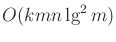time. More generally, ifis bounded byalgebraic patches of constant maximum degree, then a vertical decomposition method solves the motion planning problem within a single connected component ofin time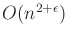.

Steven M LaValle 2020-08-14